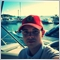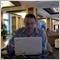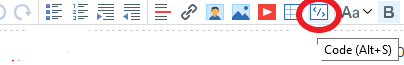# Increase width of COG line91

```#property indicator_chart_window
#property indicator_buffers 5
#property indicator_color1 RoyalBlue
#property indicator_color2 LimeGreen
#property indicator_color3 LimeGreen
#property indicator_color4 Goldenrod
#property indicator_color5 Goldenrod
//-----------------------------------
extern int bars_back = 100;
extern int m = 2;
extern int i = 0;
extern double kstd = 2.0;
extern int sName = 1102;
//----------------------
double fx[], sqh[], sql[], stdh[], stdl[];
double ai[10,10], b, x, sx;
double sum;
int    ip, p, n, f;
double qq, mm, tt;
int    ii, jj, kk, ll, nn;
double sq, std;
//*******************************************
int init()
{
IndicatorShortName("Center of Gravity");
SetIndexStyle(0, DRAW_LINE, 2 );
SetIndexBuffer(0, fx);
SetIndexBuffer(1, sqh);
SetIndexBuffer(2, sql);
SetIndexBuffer(3, stdh);
SetIndexBuffer(4, stdl);
p = MathRound(bars_back);
nn = m + 1;
ObjectCreate("pr" + sName, 22, 0, Time[p], fx[p]);
ObjectSet("pr" + sName, 14, 159);
return(0);
}
//----------------------------------------------------------
int deinit()
{
ObjectDelete("pr" + sName);
}
//**********************************************************************************************
int start()
{
int mi;
//-------------------------------------------------------------------------------------------
ip = iBarShift(Symbol(), Period(), ObjectGet("pr" + sName, OBJPROP_TIME1));
p = bars_back;
sx = p + 1;
SetIndexDrawBegin(0, Bars - p - 1);
SetIndexDrawBegin(1, Bars - p - 1);
SetIndexDrawBegin(2, Bars - p - 1);
SetIndexDrawBegin(3, Bars - p - 1);
SetIndexDrawBegin(4, Bars - p - 1);
//----------------------sx-------------------------------------------------------------------
for(mi = 1; mi <= nn * 2 - 2; mi++)
{
sum = 0;
for(n = i; n <= i + p; n++)
{
sum += MathPow(n, mi);
}
sx[mi + 1] = sum;
}
//----------------------syx-----------
for(mi = 1; mi <= nn; mi++)
{
sum = 0.00000;
for(n = i; n <= i + p; n++)
{
if(mi == 1)
sum += Close[n];
else
sum += Close[n] * MathPow(n, mi - 1);
}
b[mi] = sum;
}
//===============Matrix=======================================================================================================
for(jj = 1; jj <= nn; jj++)
{
for(ii = 1; ii <= nn; ii++)
{
kk = ii + jj - 1;
ai[ii, jj] = sx[kk];
}
}
//===============Gauss========================================================================================================
for(kk = 1; kk <= nn - 1; kk++)
{
ll = 0; mm = 0;
for(ii = kk; ii <= nn; ii++)
{
if(MathAbs(ai[ii, kk]) > mm)
{
mm = MathAbs(ai[ii, kk]);
ll = ii;
}
}
if(ll == 0)
return(0);

if(ll != kk)
{
for(jj = 1; jj <= nn; jj++)
{
tt = ai[kk, jj];
ai[kk, jj] = ai[ll, jj];
ai[ll, jj] = tt;
}
tt = b[kk]; b[kk] = b[ll]; b[ll] = tt;
}
for(ii = kk + 1; ii <= nn; ii++)
{
qq = ai[ii, kk] / ai[kk, kk];
for(jj = 1; jj <= nn; jj++)
{
if(jj == kk)
ai[ii, jj] = 0;
else
ai[ii, jj] = ai[ii, jj] - qq * ai[kk, jj];
}
b[ii] = b[ii] - qq * b[kk];
}
}
x[nn] = b[nn] / ai[nn, nn];
for(ii = nn - 1; ii >= 1; ii--)
{
tt = 0;
for(jj = 1; jj <= nn - ii; jj++)
{
tt = tt + ai[ii, ii + jj] * x[ii + jj];
x[ii] = (1 / ai[ii, ii]) * (b[ii] - tt);
}
}
//===========================================================================================================================
for(n = i; n <= i + p; n++)
{
sum = 0;
for(kk = 1; kk <= m; kk++)
{
sum += x[kk + 1] * MathPow(n, kk);
}
fx[n] = x + sum;
}
//-----------------------------------Std-----------------------------------------------------------------------------------
sq = 0.0;
for(n = i; n <= i + p; n++)
{
sq += MathPow(Close[n] - fx[n], 2);
}
sq = MathSqrt(sq / (p + 1)) * kstd;
std = iStdDev(NULL, 0, p, MODE_SMA, 0, PRICE_CLOSE, i) * kstd;
for(n = i; n <= i + p; n++)
{
sqh[n] = fx[n] + sq;
sql[n] = fx[n] - sq;
stdh[n] = fx[n] + std;
stdl[n] = fx[n] - std;
}
//-------------------------------------------------------------------------------
ObjectMove("pr" + sName, 0, Time[p], fx[p]);
//----------------------------------------------------------------------------------------------------------------------------
return(0);
}
//==========================================================================================================================   ```

Can somebody help me to inscrease the width of the lines to 2?Moderator
113956

When you post code please use the CODE button (Alt-S)!91

Sergey Golubev:

Thanks, it's updated91

I solved with

``` SetIndexStyle(0, DRAW_LINE, EMPTY,2, indicator_color1);
SetIndexStyle(1, DRAW_LINE, EMPTY,2, indicator_color2);
SetIndexStyle(2, DRAW_LINE, EMPTY,2, indicator_color3);
SetIndexStyle(3, DRAW_LINE, EMPTY,2, indicator_color4);
SetIndexStyle(4, DRAW_LINE, EMPTY,2, indicator_color5);```